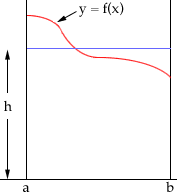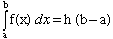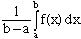Name: Esther Who is asking: Parent Level: Secondary Question: On a recent Calculus paper my son was unsure if he answered two questions correctly. This is one of them; we'd sure appreciate a clarification. Thanks. The average value of a continuous function y = f(x) on the interval [a,b] is given by ________________? Hi Esther, I think of the "average value of a continuous function" in the following way. Imagine that you have the graph of y = f(x) between a and b as in the diagram below.The average value of the function f(x) is the height h so that the area under the graph of y = f(x) from a to b is the same as the area of the rectangle on the same base with height h. That isThus the average value of y = f(x) from a to b isCheers, Harley Go to Math Central